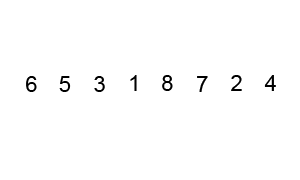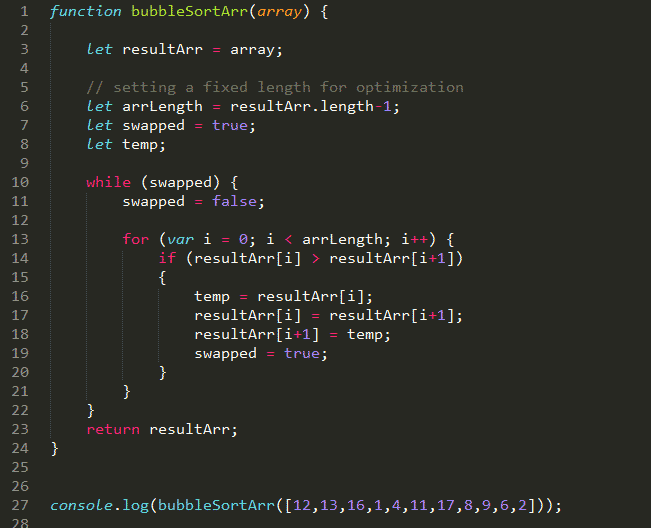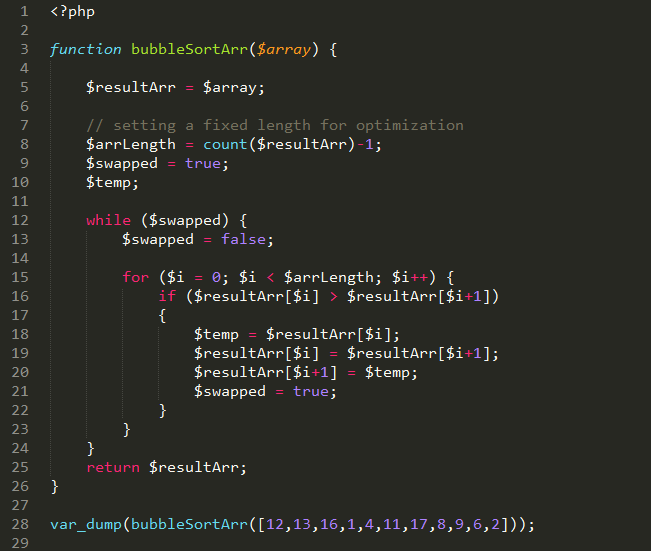• Time complexity: O(n2)

Bubble sort is one of the easiest sorting algorithms to implement. The name is derived from the process of sorting based on gradually “bubbling” (or rising) values to their proper location in the array. The bubble sort repeatedly compares adjacent elements of an array as in the following picture:The first and second elements (4 and 2) are compared and swapped if out of order. Then the second and third elements (4 and 5) are compared and swapped if out of order and so on. The algorithm consists of two loops. In each iteration a single value is set in the right place. This sorting process continues until the last two elements of the array are compared and swapped if out of order (the last iteration doesn’t swap any values).• JavaScript implementation example:[source_code]

• PHP implementation example:[source_code]